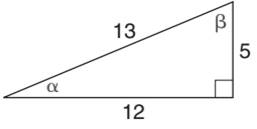Chapter 11.1, Problem 1E### Elementary Geometry for College St...

6th Edition
Daniel C. Alexander + 1 other
ISBN: 9781285195698

#### Solutions

Chapter
Section### Elementary Geometry for College St...

6th Edition
Daniel C. Alexander + 1 other
ISBN: 9781285195698
Textbook Problem
1 views

# In Exercises 1 to 6, find sin     α and sin     β for the triangle shown.To determine

To find:

The angle sinαandsinβ

Explanation

In a right angle triangle,

1) The side PQ, which is opposite to the right angle PRQ is called the hypotenuse.

(The hypotenuse is the longest side of the right triangle.)

2) The side RQ is called the adjacent side of angle θ.

3) The side PR is called the opposite side of angle θ.

Hence, the sine ratio for an acute angle is

Ratio =oppositehypotenuse

Given:

The diagram is,

For angle α, the opposite side is 5 and hypotenuse side is 13

### Still sussing out bartleby?

Check out a sample textbook solution.

See a sample solution

#### The Solution to Your Study Problems

Bartleby provides explanations to thousands of textbook problems written by our experts, many with advanced degrees!

Get Started

#### (a) 715and121 (b) 38 and 57 (c) 2.6 and 1.8

Precalculus: Mathematics for Calculus (Standalone Book)

#### Simplify: 5251155

Elementary Technical Mathematics

#### In Exercises 49-62, find the indicated limit, if it exists. 55. limx1xx1

Applied Calculus for the Managerial, Life, and Social Sciences: A Brief Approach

#### In problems 45-62, perform the indicated operations and simplify. 58.

Mathematical Applications for the Management, Life, and Social Sciences

#### True or False:

Study Guide for Stewart's Multivariable Calculus, 8th

#### Find if , y = sin 2t.

Study Guide for Stewart's Single Variable Calculus: Early Transcendentals, 8th